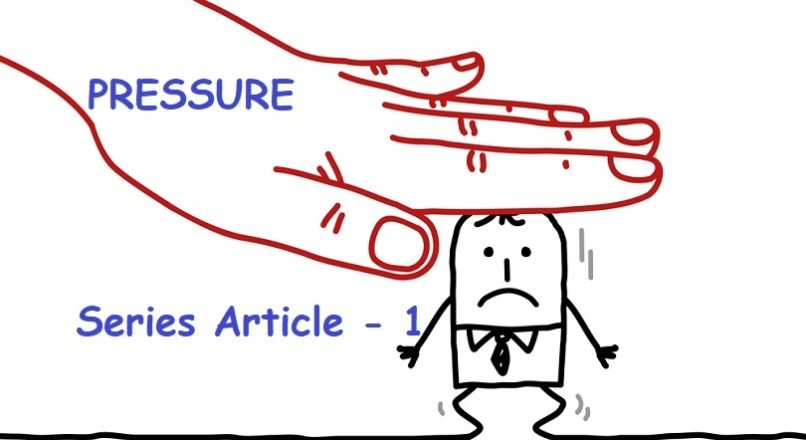## Register Now

Join now to get your problems solved with ease. Register with Email. Feel free to WhatsApp all issues on 8294600829.# Pressure

Pressure is defined as the force applied on a unit area; it is represented by the symbol P. The unit of pressure is Pascal or Newton per meter squared.

P= Force/Area

In this article we will try to understand pressure qualitatively and quantitatively. At the end of this read you should be able to appreciate cause of pressure, atmospheric pressure, types of pressure and variation of pressure in static fluid.

#### What causes pressure?

When a given amount of gas is put inside a closed container, it exerts pressure on the walls of the container. We know that gaseous molecules keep moving randomly hence they collide with the wall, causing change in momentum (p=mv) hence force (dp/dt) is exerted on the walls. For increasing the force we can increase the frequency of collision. It can be achieved using two mechanisms,

1. i) Increase the temperature of the gas which will increase the thermal energy of molecules. The particles will have more momentum (velocity increases) hence will exert more force on walls.
2. ii) Increasing density of the gas will result in more number of collisions i.e frequency of collision will increase so pressure will go up.

Pressure =f(Temperature, Density)                 (Macroscopically)

#### Calculating pressure

P = F/A

P = Mg/A = ρVg/A = ρAhg/A = ρgh

P= pressure

M=mass= Density (× Volume (V)

A= area

g= Acceleration due to gravity

We observe that pressure in a fluid at rest depends directly on density of fluid, height of fluid above the point under consideration and acceleration due to gravity.

Pressure increase with the depth but pressure on a given particle in a static fluid is same in all directions, this conclusion can easily be drawn from the fact that every particle in a state of static equilibrium or rest.F1=F2=F3=F4

#### Atmospheric pressure

Earth is enveloped with a layer of air or atmosphere which extends up to around 100 kilometers. The pressure exerted by the atmosphere on things is called atmospheric pressure. Here we will treat air as a fluid. It is clear from the previous discussion that pressure on the surface of the earth will be maximum as compared to on mountains.1Bar=101325 Pascals

The steep decrease in pressure with altitude is accounted for by the decrease in density of air as the altitude increases. This decrease in pressure is responsible for slow cooking of food on mountains. (Think!)

#### Absolute pressure and gauge pressureAbsolute pressure is measured taking the absolute zero or complete vacuum (0 bar) as reference.

When pressure is higher than the local atmospheric pressure usually 1 Bar then we use the word gauge pressure.

Absolute pressure = Local atmospheric pressure + Gauge pressure

When pressure is lower than the local atmospheric pressure then we use the word vacuum pressure.

Absolute pressure = Local atmospheric pressure- vacuum pressurePressure at point D =ρgh2

Pressure at point B= ρgh1

Pressure at point C= ρgh1

We observe that;

PD> PB and PD> PC

This can be attributed to the fact that h1 is less than h2, we must also note that it is only valid for points in same fluid (Density).

We also understand that for a given liquid the pressure at same horizontal level remains same or pressure only varies vertically. This fact will be used to solve almost all questions related to pressure calculation and manometry.

In next article we shall discuss numerical on manometry and types of pressure measuring devices.

Thanks for learning at Kailasha Foundation – Fun & Learn Portal.

I am pursuing mechanical engineering at the national institute of science and technology. I belong to Darbhanga, Bihar. My fields of interest are Thermodynamic analysis, Astronomy, Astrophysics.

## Comments ( 2 )

1.Great article for basics. The question, why does pressure increase with depth, could have been explained better (weight of fluid above increases with depth).

2. Wonderfully explained ….

error: